# KSEEB Solutions for Class 9 Maths Chapter 13 Surface Area and Volumes Ex 13.2

In this chapter, we provide KSEEB SSLC Class 9 Maths Chapter 13 Surface Area and Volumes Ex 13.2 for English medium students, Which will very helpful for every student in their exams. Students can download the latest KSEEB SSLC Class 9 Maths Chapter 13 Surface Area and Volumes Ex 13.2 pdf, free KSEEB SSLC Class 9 Maths Chapter 13 Surface Area and Volumes Ex 13.2 pdf download. Now you will get step by step solution to each question.

## Karnataka Board Class 9 Maths Chapter 13 Surface Area and Volumes Ex 13.2

(If values are not given for ‘π’ Assume π = 227)

Question 1.
The curved surface area of a right circular cylinder of a height 14 cm is 88 cm2. Find the diameter of the base of the cylinder.
Solution:
Lateral surface area of cylinder which has height 14 cm is 88 cm2.
Diameter of bottom of cylinder, d = ?
h = 14 cm, L.S.A. = 88 cm2, d = ?
Lateral surface area of cylinder, L.S.A.
L.S.A.= 2πrh = 88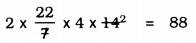88 × r = 88
∴ r = 8888 ∴ r = 1 cm.
∴ Diameter, d = 2r =2 × 1 = 2 cm.

Question 2.
It is required to make a closed cylinderical tank of height 1 m and base diameter 140 cm from a metal sheet. How many square metres of the sheet are required for the same ?
Solution:
Height of cylindrical water tank, h = 1 m
Diameter of bottom, d = 140 cm.
h = 1 m, d = 140 cm,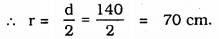r = 0.7 m.
Area of metal sheet required to prepare water tank, A
A = 2πr (r + h)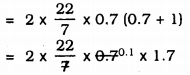= 44 × 0.1 × 1.7
= 4.4 × 1.7
A = 7.48 m2.

Question 3.
A metal pipe is 77 cm long. The inner diameter of a cross section is 4 cm, the outer diameter being 4.4 cm (see Fig. 13.11). Find its
(i) inner curved surface area,
(ii) outer curved surface area,
(iii) total surface area.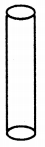Solution:
Length of metal pipe, l = 77 cm,
h = 77 cm.
Inner diameter = 4 cm, ∴ r1 = 2 cm.
Outer diameter= 4.4 cm. ∴ r2 = 2.2 cm.
(i) Inner surfce area of pipe (C.S.A) = 2πr1h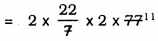= 2 × 22 × 2 × 11
= 968 cm2.
(ii) Outer surface area of pipe (C.S.A) = 2πr2h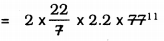= 2 × 22 × 2.2 × 11
= 44 × 24.2
= 1064.8 cm2.
(iii) Total Surface area of Pipe (T.S.A.) = Inner CSA + Outer CSA + Area of circular ring.
= 2πr1h + 2πr2h + (2πr22h – 2πr12h)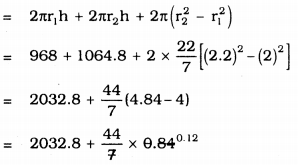= 2032.8 + 5.28
∴ T.S.A. = 2038.08 cm2.

Question 4.
The diameter of a roller is 84 cm and its length is 120 m. It takes 500 complete revolutions to move once over to level a playground. Find the area of the playground in m2.
Solution:
Roller is in the form of cylinder.
Diameter, d = 84 cm,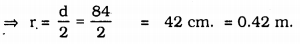l = h = 120 cm ⇒ 1.2 m.
∴ Curved surface area of Roller = 2 πrh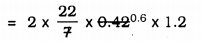= 44 × 0.072
= 3.168 m2
∴ Area of 1 round of roller = 3.168 m2.
Area of 500 rounds … ? …
= 3.168 × 500
= 1584 m2.
∴ Total area of a playground is 1584 m2.

Question 5.
A cylindrical pillar is 50 cm in diameter and 3.5 m in height. Find the cost of painting the curved surface of the pillar at the rate of Rs. 12.50 per m2.
Solution:
Diameter of cylindrical pole, d = 50 cm.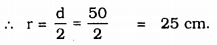∴ r = 0.25 m
height of pole, h = 3.5 m.
Curved surface area of pole = 2πrh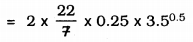= 44 × 0.25 × 0.5
= 5.5 m2.
Cost of painting for 1 m2 is Rs. 12.50,
Cost of painting for 5.5 m2 is … ? …
= 5.5 × 12.50
= Rs.68.75.

Question 6.
Curved surface area of a right circular cylinder is 4.4 m2. If the radius of the base of the cylinder is 0.7 m, find its height.
Solution:
Curved surface arc of cylinder = 4.4 m2
radius, r = 0.7 m height, h = ?
C.S.A. of cylinder, A= 2πrh
2πrh = 4.4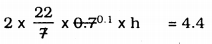44 × 0.1 × h = 4.4
4.4 h = 4.4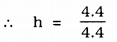∴ h = 1 m.

Question 7.
The inner diameter of a circular well is 3.5 m. It is 10 m deep. Find
(i) its inner curved surface area.
(ii) the cost of plastering this curved surface at the rate of Rs. 40 per m2.
Solution:
Inner diameter of circular well, d = 3.5 m.
Depth of the well – height, h = 10 m.
(i) Inner surfacer area of circular well, C.S.A. = 2πrh
C.S.A. = 2πrh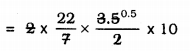= 22 × 0.5 × 10
= 110 m2
(ii) Cost of plastering of area 1 m2 is Rs. 40
Cost of plastering 110 m2… ? …
= 110 × 40
= Rs. 4400.

Question 8.
In a hot water heating system, there is a cylindrical pipe of length 28 m and diameter 5 cm. Find the total radiating surface in the system.
Solution:
Diameter of cylindrical pipe, d = 5 cm
= 0.5 m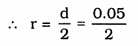Length of pipe = height, h = 28 m.
Curved surface area of pipe = ?
C.S.A.= 2πrh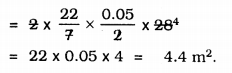Question 9.
Find
(i) The lateral or curved surface area of a closed cylindrical petrol storage tank that is 4.2 m in diameter and 4.5 m high.
(ii) how much steel was actually used, if 112 of the steel actually used was wasted in making the tank.
Solution:
(i) Petrol tank is in the form of cylinder.
Diameter, d = 4.2 m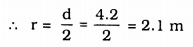h = 4.5 m
TSA of petrol tank = 2πr(r + h)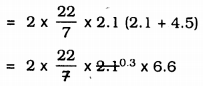= 44 × 0.3 × 6.6
= 87.12 m2
(ii) ∴ Area of iron required for manufacturing tanker = 87.12 m2.
If iron used is 1 sq. m then there is a loss of 112.
Iron required except loss = 1−112
= 1112 m2
Area of 1112 m2 is 87.12 m2.
Area of 1 m2 … ? …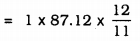= 95.04 m2.

Question 10.
In Fig. 13.12, you see the frame of a lampshade. It is to be covered with a decorative cloth. The frame has a base diameter of 20 cm and height of 30 cm. A margin of 2.5 cm is to be given for folding it over the top and bottom of the frame. Find how much cloth is required for covering the lampshade.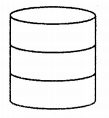Solution:
Diameter of cylindrical frame, d= 20 cm,
∴ r = 10 cm.
height, h = 30 cm.
For folding 2.5 cm is required.
∴ Total height = 30 + 2.5 + 2.5
= 35 cm.
Curved surface area of cloth covered, A
A= 2πrh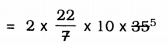= 44 × 50
= 2200 cm2.

Question 11.
The students of a Vidyalaya were asked to participate in a competition for making and decorating penholders in the shape of a cylinder with a base, using card-board. Each penholder was to be of radius 3 cm and height 10.5 cm. The Vidyalaya was to supply the competitors with cardboard. If there were 35 competitors, how much cardboard was required to be bought for the competition?
Solution:
Penholder is in the shape of a cylinder, which one of the edges is open and another is closed.
radius, r = 3 cm, height, h = 10.5 cm.
Total cardboard required by one Competitor: = Curved Surface Area + Area of bottom = 2πrh + πr2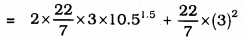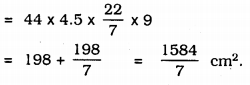∴ Total cardboard required for 35 competitors is :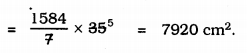All Chapter KSEEB Solutions For Class 9 Maths

—————————————————————————–

All Subject KSEEB Solutions For Class 9

*************************************************

I think you got complete solutions for this chapter. If You have any queries regarding this chapter, please comment on the below section our subject teacher will answer you. We tried our best to give complete solutions so you got good marks in your exam.

If these solutions have helped you, you can also share kseebsolutionsfor.com to your friends.

Best of Luck!!